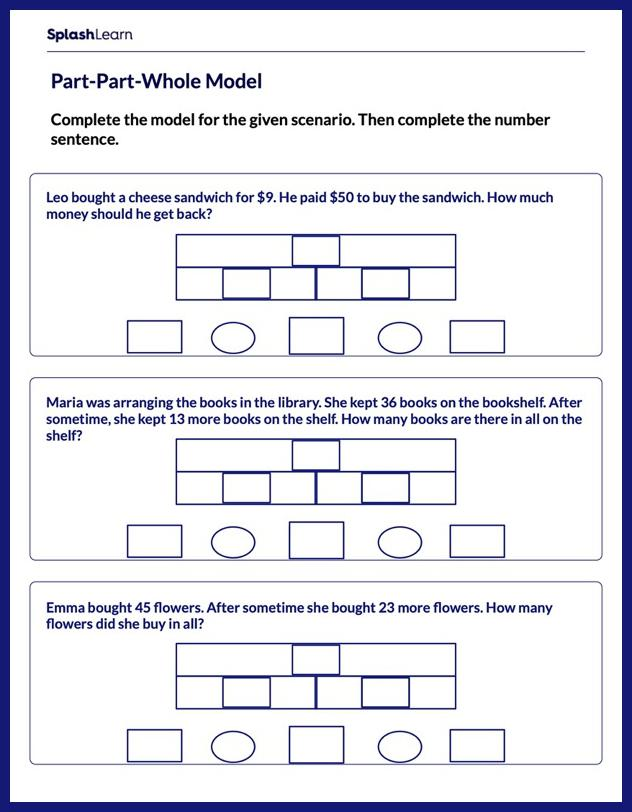# Model the Scenario and Complete the Equation Worksheet

Home > Model the Scenario and Complete the EquationHelp your child become an expert in word problems with this worksheet. Young learners will make connections between math and the real world as they solve a set of addition and subtraction word problems involving a variety of scenarios. In these problems, they comprehend the scenarios and represent the situation using models. Here the rigor is beautifully balanced by asking students to work with 2-digit numbers.